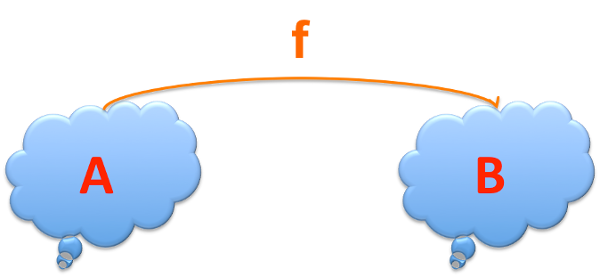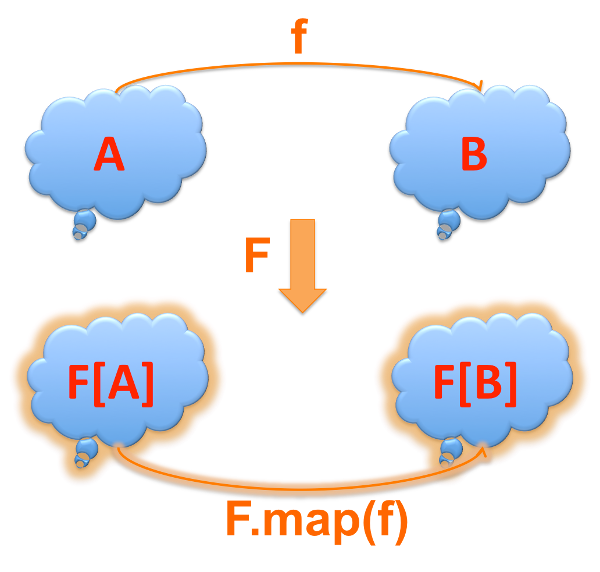## From function to functor and onto monads Pub

Some more details, to follow up on our first encounter with monads in Monads shmonads and functional programming... we'll work on moving conceptually from function to functor.

The functor is the first step in comprehending monads, for me - it lifts a simple function `f : A => B` into something higher level, `F[A] => F[B]`.

Here’s my conceptual progression: we start on our monadic journey from functions, so we have a function from A to B

`f: A => B`A function transforms As into Bs

Then, we have a weird Functor let’s call it `F` and it can “lift” a given function f to work on the entire set: `F.map(f)` will take a function f mapping elements of A into elements of B and return a contraption that will turn an entire set `F[A]` into a `F[B]`, the signature of the contraption being `F[A] => F[B]`

Hence the signature of the contraption generator being:

``````// defined outside of F
object FFunctor[F] {
def unit[A]  (a : A*) : F[A]
def map[A,B]  (f : A => B) : F[A] => F[B]
}
``````

OR, if defined as a member of F

``````class F[A] {
def this (a:A*) = {}
def map[B]  (f : A => B) : F[B]
}
``````

Notice the difference… the second one, since it’s a member of a `F[A]` already, it will return the `F[B]` directly, bypassing the contraption (or so you'd think, until you realize that many collections are lazy... so in reality you are getting something that will eventually get you an F[B], as needed).

``````// version 1
val x : F[A] = FFunctor.unit(a1, a2)
val contraption : F[A] => F[B] = FFunctor.map (f)
val y : F[B] = contraption (x)

// version 2
val x : F[A] = new F(a1, a2)
val y : F[B] = x.map (f)
``````

Now instead of `F` think `List` or `HashMap` or `Option`… they’re all Functors (i.e. contraptions).A functor takes an A, f and B and transforms them all

As you can see in the diagram above, the two components of the `F` functor are:

• `A => F[A]` and `B => F[B]` respectivelly, which is traditionally called `unit()`
• `A => B => F[A] => F[B]` which is traditionally called `fmap()` or in scala collections, replaced by a method form called `map()`.

When using the method form, the role of `unit` is played by the type constructor, i.e. `List(a)` just took an `a:A` and made it a `List[A]`. This is why there's no method called `unit` on List.

The first form is customary in languages that decouple the structures from their Functors, while the second is typical in OO languages.

So again, to recap, starting from a simple function, which is a transformation from A to B:

`f : A => B`

a Functor `F` will lift that to work on weird things like `F[_]` the resulting thing will be a functor, i.e. something that transforms an `F[A]` into an `F[B]`:

`F[A] => F[B]`

Now you're ready to read the more precise definition of a Functor in wikipedia.

## Some examples

Let's drive it home with some actual examples.

Here's a transformation from String to Int `f : String => Int` and a strange Functor called `List` which we'll use to transform lists `List[String] => List[Int]` :

``````def f (a:String) : Int = a.toInt

val listB : List[Int] = List[String](“1”, “2”).map(f)   // == List(1,2)
``````

Here's another, a transformation from Student to Int and another strange Functor called `Seq` :

``````case class Student(name:String, age:Int)

def f (a:Student) : String = a.name

val listNames : Seq[String] = Seq[Student](Student(“John”, 21), Student("Mary", 22).map(f)   // == Seq("John", "Mary")
``````

Here's a way to use all this:

``````val ages = db.findAll[Student]("class" -> "2016").map(_.age)
val averageAge = ages.sum / ages.size
``````

We couldn't care less what findAll returns, as long as it has a map() method (i.e. it is a functor). `sum` is a `fold` as in

`def sum = this.fold(0)(_ + _)`

... and that's where the Functors stop. I think this is a big step into the abstractions of category theory and making functional programming actually useful. We'll make the smaller step to monads soon.

## Functional composition

The basic rule of functors refers to composition:

`F[f o g] = F[f] o F[g]`

... basically, a proper functor will obey this rule!

## Architectural significance

This stuff is not relevant just for a sub-culture of "functional programming", but to the much larger sub-culture of "architecting it the wrong way".

Too many times I see architectures and designs that add unnecessary complexity in component / framework / product design because the architects lack a basic understanding of functional composition principles.

Functional programming is not only useful because we now have multiple cores and stateless web servers and reactive I/O libraries (and single-tasking node/JS) but also because it leads to a compositional architectural style that is completely uncluttered.

A clean, functional approach to composition would help all the way to architecting product lines and assigning work to teams and resources more efficiently.

Did someone in the gallery yell microservices? If that's your `f` though... where's your... `F`? Food for thought!